# Basic Arithmetic : Reciprocals

## Example Questions

### Example Question #1 : Manipulation Of Fractions

What is the reciprocal of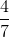?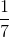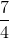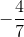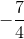Explanation:

To get the reciprocal of a fraction, you simply switch the numerator and the denominator.

In our case our numerator isand our denominator is.

Sobecomes.

### Example Question #1 : Manipulation Of Fractions

Compute: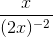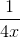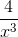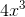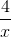Explanation:

We will need to rewrite this in order to eliminate the negative exponent in the problem.

Because the denominator has a negative exponent, that is the same as having a positive exponent in the numerator. Therefore we can rewrite the problem as follows and then multiply.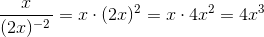### Example Question #1 : Manipulation Of Fractions

Evaluate: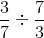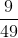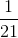Explanation:

To divide a term by a fraction, take the reciprocal of the fraction.

Then mutiply both terms.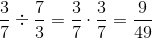### Example Question #1 : Manipulation Of Fractions

What is the sum of the reciprocal ofand?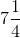Explanation:

To find the reciprocal of a fraction, flip the numerator and the denominator.

Thus, the reciprocal ofis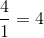.

Then we need to find the sum of 4 and 7, which is 11.

### Example Question #1 : Manipulation Of Fractions

What is the reciprocal of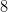multiplied by the reciprocal of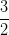?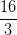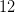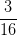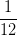Explanation:

To find the reciprocal of a fraction, we simply need to switch the numerator and the denominator: for example, the reciprocal of a fraction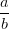is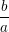.

With integers, it helps to remember that all integers are really fractions with a denominator of: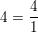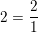, and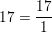The reciprocals of these numbers are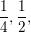and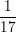respectively.

Therefore, to solve the problem, we first need to find the reciprocals ofand. If we keep in mind that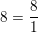, we can determine that the reciprocals are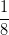and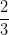, respectively. The product of these two numbers is: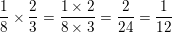is our final answer.

### All Basic Arithmetic Resources